# Class VIII-Math-Exponents and Powers

##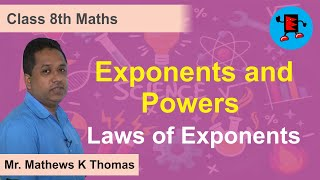By Extraminds

Class VIII-Math-Exponents and Powers# Maths Powers part 5 (Laws of Exponents) CBSE Class 8 Mathematics VIII

##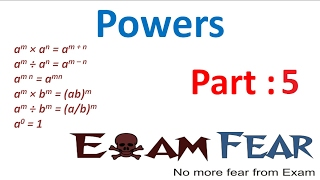By ExamFear Education

Maths Powers part 5 (Laws of Exponents) CBSE Class 8 Mathematics VIII# Powers and exponents

##By MathPlanetVideos

Rewrite the expressions 2⋅2⋅2 x⋅x⋅x⋅x⋅x 3^4 x^3# Simplifying a radical expression using rational exponents

##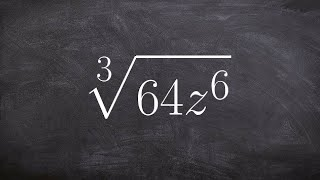By Brain McLogan

Learn how to simplify rational powers using the power and the product rules. There are some laws of exponents which might come handy when simplifying expressions with exponents. Some of the laws include the product law which states that the product of numbers/expressions having the same base is equivalent to taking one of the base with the sum of the original exponents as the new exponent. The power law says that when an expression with an exponent is raised to another exponent that it is equivalent to the expression with the product of the original exponents as the new exponent. Knowledge of these exponent laws will help you in simplifying power expressions using the power rule and the quotient rule.# Algebra 2 - Rational Exponents

##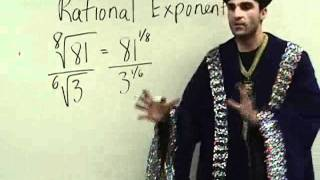By Yaymath

Join the mathemagician's adventure into simplifying expressions with different exponents. Fraction exponents, negative exponents, and roots of varying powers. YAY MATH!# Powers and Exponents

##By Anywhere Math# Power Rule | Multiplying Exponents | MathHelp.com

##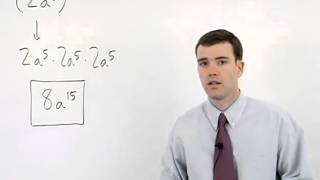By MathHelp.com# What is an Exponent & Powers of 10? - 

##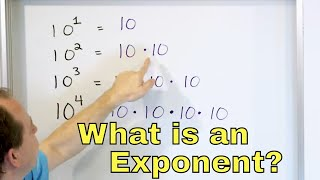By Math and Science

Quality Math And Science Videos that feature step-by-step example problems!# 01 - Simplify Rational Exponents (Fractional Exponents, Powers & Radicals) - Part 1

##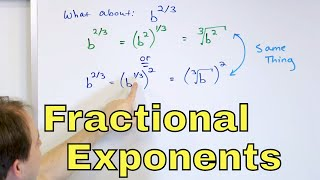By Math and Science

Quality Math And Science Videos that feature step-by-step example problems!# ACT Prep - Exponents

##By Dr.Steeve Warner

ACT Prep - Exponents# Exponential form to find complex roots | Imaginary and complex numbers | Precalculus | Khan Academy

##By Khan Academy# Finding the Equation of an Exponential Function

##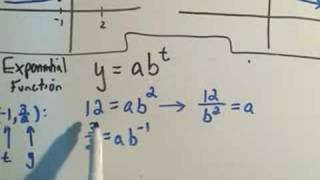By PatrickJMT

Finding the Equation of an Exponential Function - The basic graphs and formula are shown along with one example of finding the formula for an exponential function given two points that the function goes through.##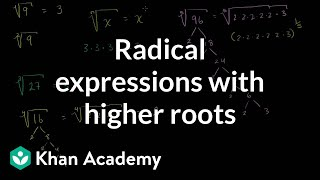By Khan Academy

Sal explains the relationship between radical notation and unit-fraction exponents, including a discussion of what the principal square root is. Then he shows how to simplify the 6th root of (64x^8) using the properties of exponents.##By Khan Academy

Sal explains what it means to take a number by a power which is a unit fraction, like 1/2, 1/3, etc.# DEPRECATED Simplifying expressions with exponents

##By Khan Academy

Exponent Properties 4# DEPRECATED Simplifying expressions with exponents

##By Khan Academy

When multiplying numbers with common base, add exponents# 7.1 Integer Exponents - Algebra 1

##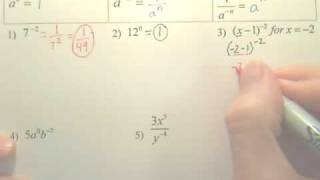By WOWmath.org

This is a video lecture course on Fundamentals of Algebra by Robb, a High School Math Teacher and includes Integers, Fractions, Decimals, Order of Operations, Combining Like Terms, Evaluating Expressions, Solving Equations, Solving Inequalities, Graphing Linear Equations, Systems of Equations and more.# DEPRECATED Simplifying expressions with exponents

##By Khan Academy

Exponent Properties 3# DEPRECATED Simplifying expressions with exponents

##By Khan Academy

Sal rewrites (r^(2/3)s^3)^2*ï¿½ï¿½ï¿½ï¿½ï¿½ï¿½ï¿½ï¿½ï¿½ï¿½ï¿½ï¿½ï¿½ï¿½ï¿½ï¿½ï¿½ï¿½(20r^4s^5), once as an exponential expression and once as a radical expression.# DEPRECATED Simplifying expressions with exponents

##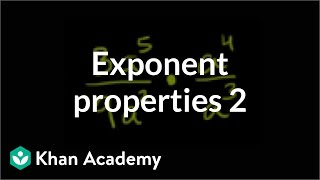By Khan Academy

Exponent Properties 2